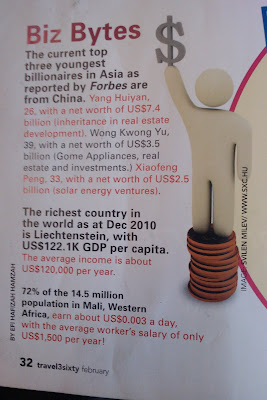## Monday, February 28, 2011

### 85% of Statistics Are Made Up On The Spot

I had a good chuckle the other day when I was caught by an example of numerical illiteracy on the part of at least two people: an author and an editor. I had to share.

I was flying with Air Asia from Banda Aceh, Indonesia to Kuala Lumpur, Malaysia. The in flight magazine isn't exactly high production value, as the airline is all about saving. Consider Air Asia to be the Ryan Air of the East. Anyways, here's the tasty treat now:I can take no issue with the first section on young billionaires as it was actually quite interesting. In the second section, I am entertained by the translation of \$122.1k GDP per capita to an average income of about \$120,000 per year. Taking the crown though, was the gem at the bottom.

"72% of the 14.5 million population in Mali, Western Africa, earn about \$0.003 a day with the average worker's salary of only US\$1,500 per year!" Now what is that supposed to mean?

Before you reach for your calculator I can tell you that \$0.003/day = \$1.10/year.
Also I can tell you that 72% of 14.5 million = 10.44 million.
And that (10.44 million people * \$1.10 per person per year ) / \$1,500 per worker per year = 7656 workers.
And that 7656/10.44 million = 0.07% employment.

Curiously I can't quite determine what I think they were going for. Anything I try to explain the numbers I see gets destroyed anyway by the strange "72% of 14.5 million". According to Wikipedia, only 43.51 million out of the 81.76 million people in Germany are employed. I suppose I could say that 53% of Germans earn about \$0 per day. By adding a dash of real workers I could make that figure \$0.003.

Marianela said...

Yeah, weird numbers. However, my quick calc does not agree with yours. See, I think your third equation either doesn’t apply or it calculates the percentage of workers included in the 72% rather than the total amount of workers in Mali. This is the way I see it.

I agree with the \$1.10 US/year, however this applies only to a 72% of the population.
Let us assume that this 72% of the population includes a certain percentage a of the total number of workers (w) and a certain percentage b of the total number of non workers (nw). Also that w+nw= 14.5 million.
With this I use the following equation to calculate the number of workers:
72%*14.5=a% * w + b% *(14.5-w).
So the number of workers would be: w=(14.44-b%*14.5)/(a%-b%)
So now you need to calculate a and b. For simplicity, and because it kind of makes sense, let’s assume that b=100, meaning that all non workers are included in this 72%. Also for the sake of the calculation, let’s assume that the remaining (1-a)% of workers compensate for the low income of this a% and make proportionallymore money. Therefore we have a% of workers is to 1.1 US\$/day as 100% of workers is to 1,500 US\$ /day.
So a%=1.1*100/1500 = 0.073%.
We replace a and b into our workers’ equation and get that the number of workers is 4.06 million, which is about 28% of the population... Mostly everyone not included in the 72%.

This makes a bit more sense, especially when Wikipedia says that "In 2007, about 48% of Malians were less than fifteen years old, 49% were 15–64 years old, and 3% were 65 and older. The median age was 15.9 years.". That means that if we assume no child labour and no senior labour, only 49% of the population is able to work, which brings the employment to 28%/49% =57%. That means that unemployment is 43%, which makes more sense also if you see other Wikipedia statements like “The population is predominantly rural (68% in 2002) and 5–10% of Malians are nomadic.” Or “Total fertility rate was 7.4 children per woman”, which leads me to believe (I might be wrong) that many women in working age do not work.

Odd number presentation non the less... all calculations will deliver wrong conclusions if the numbers are incorrect.

Paul A. Rubin said...

I'll take your word for the USD 1.10/year figure (which depends on the number of working days in a year). If 72% of the population average that, and the other 28% average about USD 5,354/year, the overall mean is USD 1,500/year.

Your point about the 14.5 million is well-taken, on two fronts. First, the CIA World Factbook estimates the population as 13.8 million as of July 2010. Second, it lists the labor force (as of 2007) at 3.24 million. (It also estimates per capita GDP at USD 1200 for the past three years, so the 1500 is again suspect.)